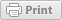Follow

# Overview

When setting up a predictive model with Leadspace, you will provided with a numeric predictive score (0-100) for each lead. It is best practice to convert these scores to different A-D buckets. This will help with prioritization, create better views/reports, campaigns, and helping sales team action based on account segments. This article will guide you on how to do this in Salesforce.

# Setup

Under Setup section go to the 'Fields' section under the Account Object or Object Manager if you are in Lightning.

Object: Account

Create the following fields:

LS Predictive Score | Field Type: Number | Length: 3 | Decimal: 0

LS Predictive Segment | Field Type: Formula (Text) |

Formula

IF(isblank(LS_Predictive_Score__c ),"", IF(LS_Predictive_Score__c >= 96, "A",IF(LS_Predictive_Score__c >= 70, "B",IF(LS_Predictive_Score__c >= 15, "C","D"))))

NOTE: The numbers in this formula can be tweaked.

Now we will copy these fields down to the Contact level. Best practice is to only copy the 'LS Predictive Segment' field as this will be easier for your team to take action, segment, etc.

Object: Contact

Create the following fields:

LS Predictive Score | Field Type: Formula (Number) | Decimal: 0 (OPTIONAL)

Formula: Account.LS_Predictive_Score__c

LS Predictive Segment | Field Type: Formula (Text) |

Formula: Account.LS_Predictive_Segment__c

This way all contacts that are tied to the account will have the predictive score and segment as the account. This will help with creating views and reports that will help your sales team prioritize which accounts to target.

Create the following fields:

LS Predictive Score | Field Type: Formula (Number) | Decimal: 0 (OPTIONAL)

Formula: Account.LS_Predictive_Score__c

LS Predictive Segment | Field Type: Formula (Text) |

Formula: Account.LS_Predictive_Segment__c

This way all Leads that are tied to the account will have the predictive score and segment as the account. This will help with creating views and reports that will help your sales team prioritize which accounts to target.